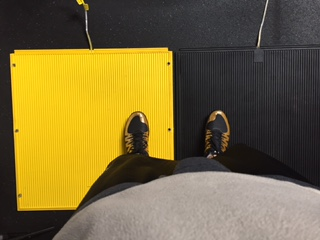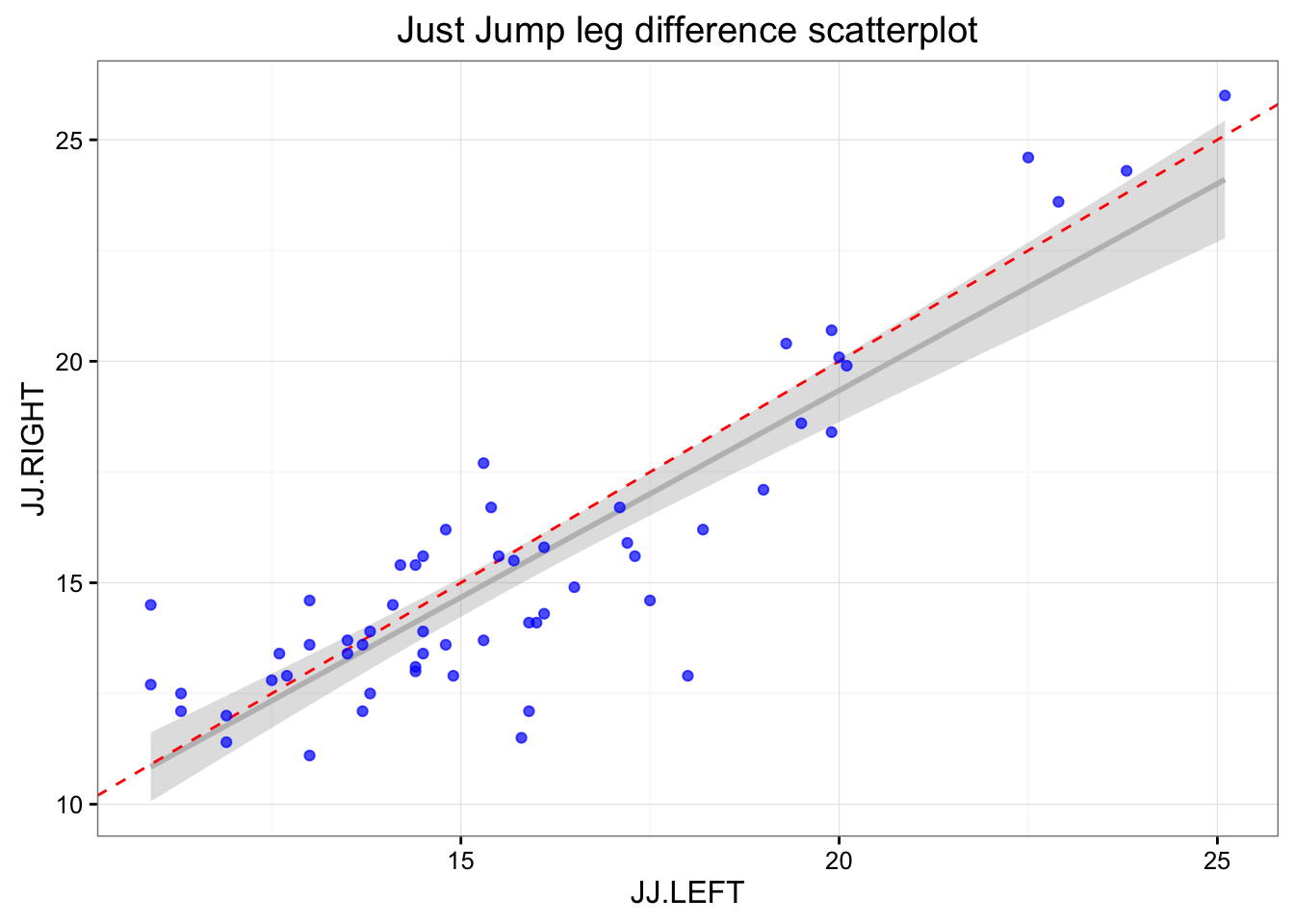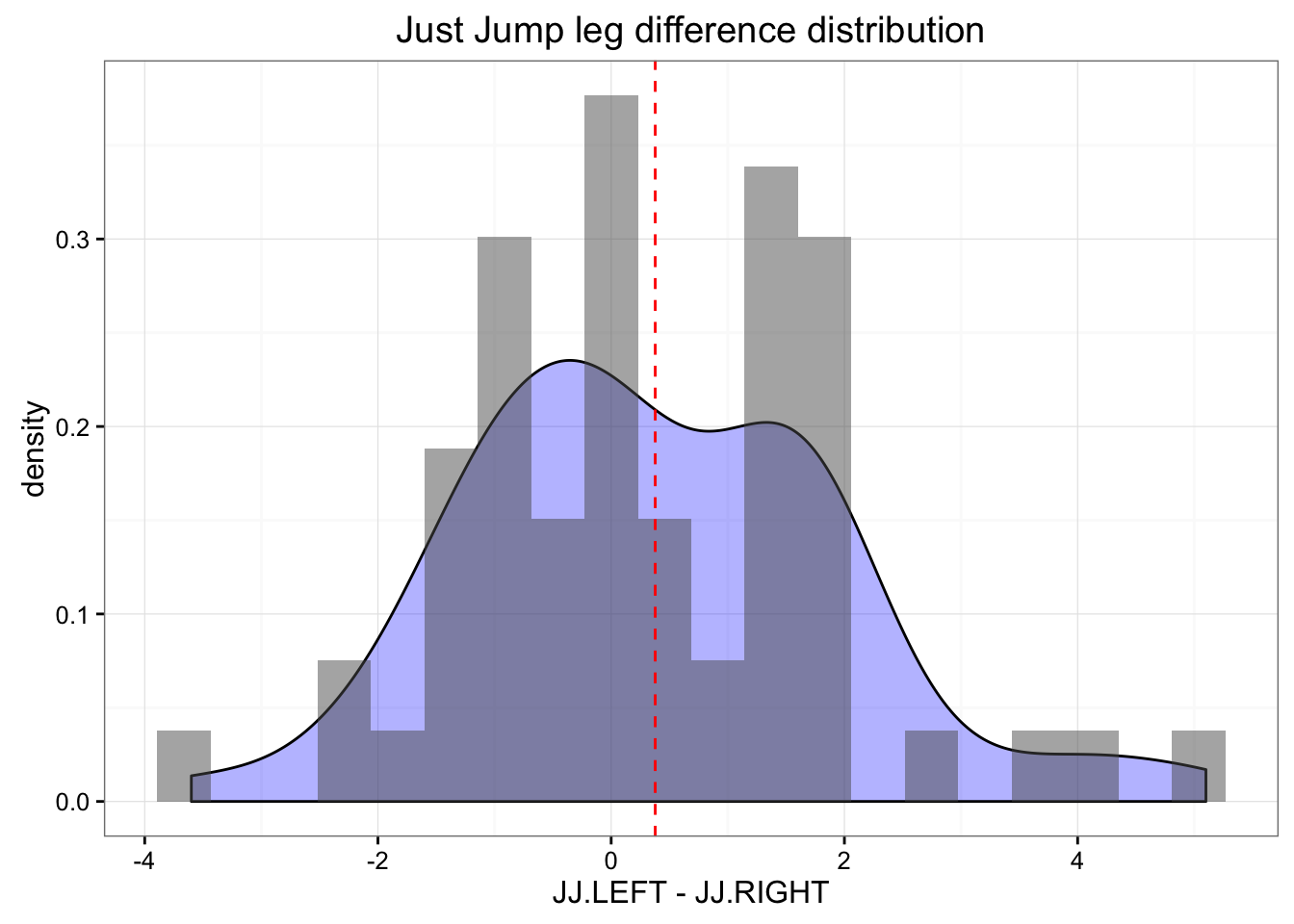## Introduction

Coaches and athletes sometime change measurement/testing equipment. The problem is that the estimates from different equipment might differ (and they usually do). There are couple of soutions to this problem - (1) disregard the old scores (in which case coaches losses precious historical data) or (2) convert the old scores to the new and ideally maintain the measurement database with as little error as possible.

Since we are going for the second option, the practical question to be answered is how big is the error, or in other words signal vs. noise? I will present one way to evaluate the error of the conversion using Smallest Worthwihle Change metric.

Friend of mine, Sam Leahey is switching from Just Jump to Smart Jump contact mats and wants to convert the older Just Jump scores to the new Smart Jump scores.

We proposed the following protocol for data collection:

1. Get couple of athletes (or all of them)
2. Put mats close to each other and place left foot on one and right foot on another (see the picture)
3. Perform the vertical jump and take the scores for each
4. Switch the legs and perfom the jump again (this should remove any leg/differences)
5. Randomize the start, to avoid any fatigue related issues
6. Multiple athletes can repreat the procedure multiple times so we get as much data points as possible

Here is the picture of the procedure:## The data

The testing data was saved to .csv file. Let’s load it and show first couple of rows

``````# Load needed libraries
suppressPackageStartupMessages(library(reshape2))
suppressPackageStartupMessages(library(caret))
suppressPackageStartupMessages(library(dplyr))
suppressPackageStartupMessages(library(rethinking))
suppressPackageStartupMessages(library(boot))

stringsAsFactors = FALSE)

``````##    Attempt    Date    Name JJ.LEFT JJ.RIGHT SJ.RIGHT SJ.LEFT
## 1        1 17.3.16     Joe    23.8     24.3    18.56   19.65
## 2        2 21.3.16     Sam    19.0     17.1    13.87   12.56
## 3        3 21.3.16    Zach    12.5     12.8    10.80    8.40
## 4        4 21.3.16    Erik    14.2     15.4    11.31   11.31
## 5        5 24.3.16     Sam    19.9     20.7    14.82   16.30
## 6        6 24.3.16  Ashton    14.5     13.4    12.51   10.57
## 7        7 24.3.16 Jillian    17.1     16.7    11.69   11.69
## 8        8 25.3.16     Joe    22.5     24.6    17.61   19.22
## 9        9 25.3.16    Zach    13.7     13.6     8.89   10.98
## 10      10 25.3.16    Erik    16.1     14.3    10.56   12.26
## 11      11 25.3.16  AidanH    14.8     13.6    10.35    9.95
## 12      12 26.3.16  Ashton    15.4     16.7    11.59   11.97
## 13      13 26.3.16    Kara    10.9     12.7     7.38    9.86
## 14      14 28.3.16     Sam    19.3     20.4    14.29   15.96
## 15      15 30.3.16     Sam    19.9     18.4    14.82   14.23``````

Let’s plot the JustJump left and right scores and SmartJump left and right scores (when left/right foot is placed on the mat).

``````# Just Jump
gg <- ggplot(jumpDataRaw, aes(x = JJ.LEFT, y = JJ.RIGHT)) +
geom_smooth(method = "lm", color = "grey", alpha = 0.3) +
geom_abline(slope = 1, color = "red", linetype = "dashed") +
geom_point(color = "blue", alpha = 0.7) +
theme_bw() +
ggtitle("Just Jump leg difference scatterplot")
print(gg)````````````gg <- ggplot(jumpDataRaw, aes(x = JJ.LEFT - JJ.RIGHT)) +
geom_density(fill = "blue", alpha = 0.3) +
geom_histogram(aes(y=..density..), bins=20, alpha = 0.5) +
geom_vline(xintercept = mean(jumpDataRaw\$JJ.LEFT - jumpDataRaw\$JJ.RIGHT),
color = "red", linetype = "dashed") +
theme_bw() +
ggtitle("Just Jump leg difference distribution")
print(gg)````````````# Smart Jump
gg <- ggplot(jumpDataRaw, aes(x = SJ.LEFT, y = SJ.RIGHT)) +
geom_smooth(method = "lm", color = "grey", alpha = 0.3) +
geom_abline(slope = 1, color = "red", linetype = "dashed") +
geom_point(color = "blue", alpha = 0.7) +
theme_bw() +
ggtitle("Smart Jump leg difference scatterplot")
print(gg)``````